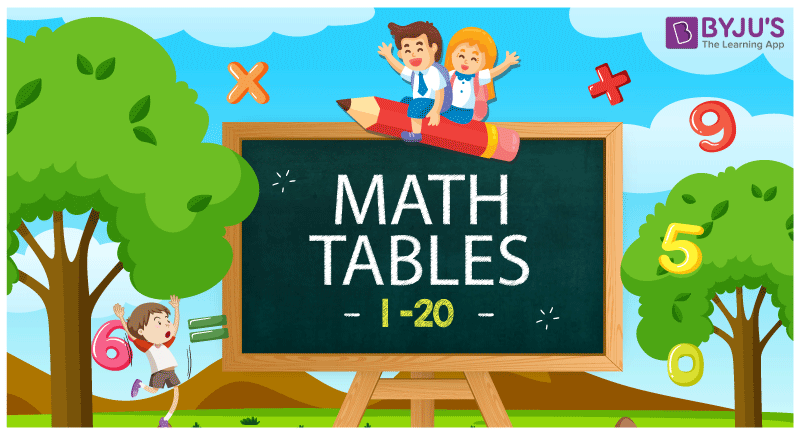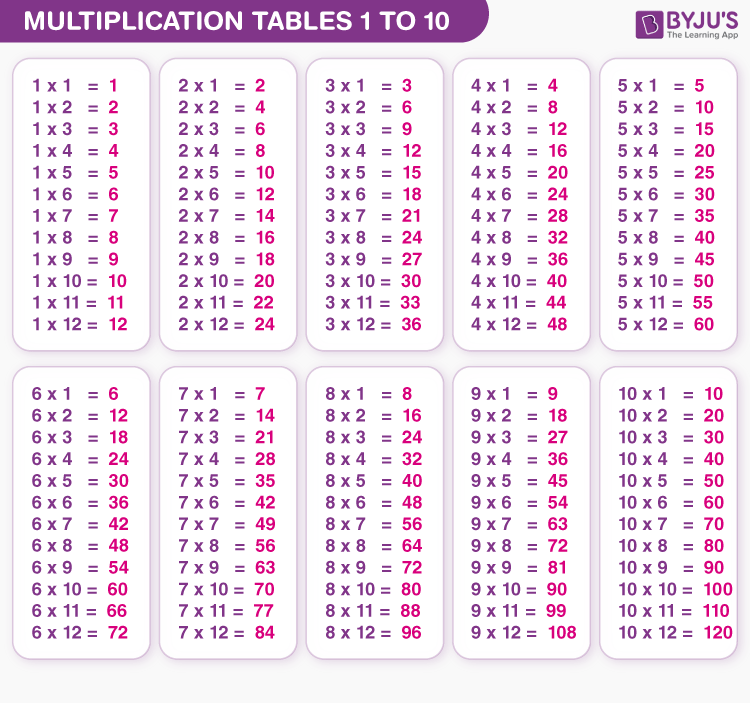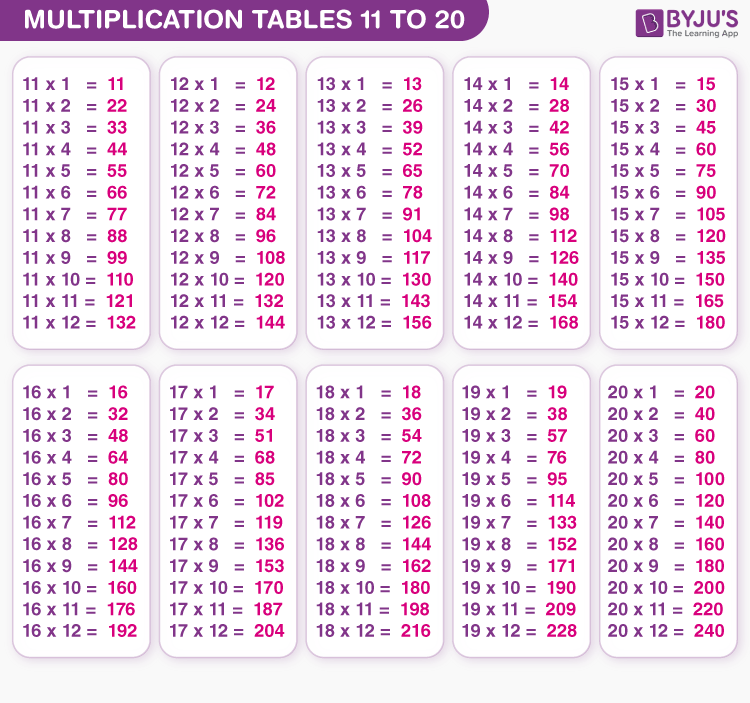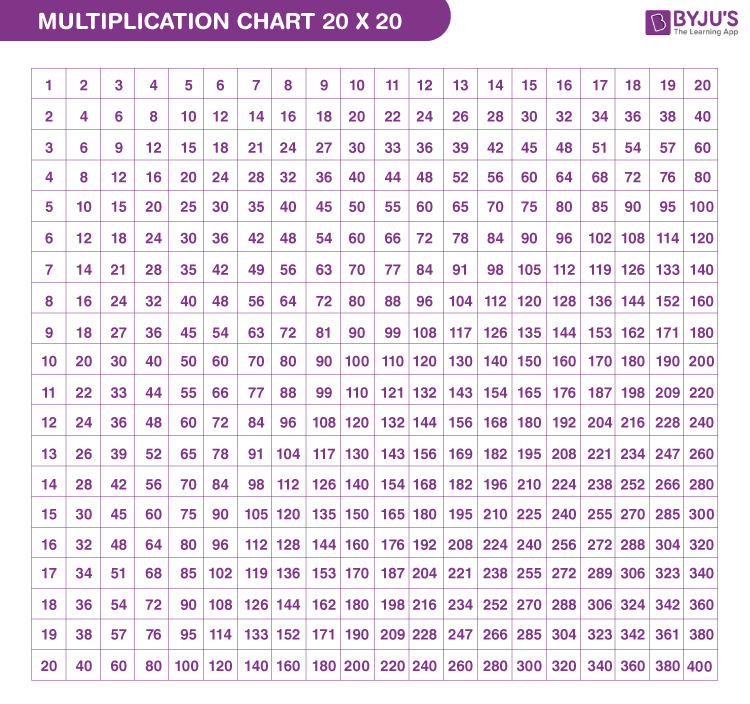# Tables 1 to 20

Tables 1 to 20 are the list of multiplication tables. The table consists of multiples of whole numbers from 1 to 20. Maths tables from 1 to 20 will help students to solve multiplication problems in a quick way. Since the table of 1 returns the same value (because all the numbers are multiplied by 1) thus we can skip it and memorise the tables 2 to 20. Students can learn the Maths tables from 1 to 10, first and then proceed with higher number multiplication tables. We have provided here tips and tricks to memorise the tables for the ease of students.

Memorizing multiplication tables 1 to 20 serves as a building block for related Maths concepts like division, fractions, long multiplication and algebra taught in elementary school. Downloadable PDFs of each table are provided at the end of the article to help students learn effortlessly and improve their problem-solving skills.## Maths Tables 1 to 20

Maths table 1 to 20 is the basis of arithmetic calculations that are most widely used in multiplication and division. Table 1 will produce the original number. Multiplication of any number with 1 results in the original number. For example, 1× ‌5 = 5, 1× ‌9 = 9 and so on.

Students are suggested to learn tables from 1 to 10, as it helps to solve the basic problems. Tables from 2 to 20 will help them to solve the complex calculations. Thus, learning multiplication tables from 1 to 20 will help students:

• To solve problems quickly
• To avoid mistakes in calculations

Note:

• Any number which is multiplied by itself gives the square of the number.
• Adding any numbers for “n” times is same as the multiplying the number with “n”. For example, adding the number 5 for 5 times results in 25. (i.e) 5+5+5+5+5 = 25. Which is also equal to 5 times 5 is 25.

The complete list of 1 to 20 tables up to 10 times is given below.

### Table 1 to 5

 Table of 1 Table of 2 Table of 3 Table of 4 Table of 5 1 ×‌ 1 = 1 2 ×‌ 1 = 2 3 × ‌1 = 3 4 × ‌1 = 4 5 × ‌1 = 5 1 ×‌ 2 = 2 2 ×‌ 2 = 4 3 × ‌2 = 6 4 × ‌2 = 8 5 × ‌2 = 10 1 × ‌3 = 3 2 × ‌3 = 6 3 × ‌3 = 9 4 × ‌3 = 12 5 × ‌3 = 15 1 × ‌4 = 4 2 × ‌4 = 8 3 × ‌4 = 12 4 × ‌4 = 16 5 × ‌4 = 20 1 × ‌5 = 5 2 × ‌5 = 10 3 × ‌5 = 15 4 × ‌5 = 20 5 × ‌5 = 25 1 × ‌6 = 6 2 × ‌6 = 12 3 × ‌6 = 18 4 × ‌6 = 24 5 × ‌6 = 30 1 × ‌7 = 7 2 × ‌7 = 14 3 × ‌7 = 21 4 × ‌7 = 28 5 × ‌7 = 35 1 × ‌8 = 8 2 × ‌8 = 16 3 × ‌8 = 24 4 × ‌8 = 32 5 × 8 = 40 1 × ‌9 = 9 2 × ‌9 = 18 3 × ‌9 = 27 4 × ‌9 = 36 5 × 9 = 45 1 × ‌10 = 10 2 × ‌10 = 20 3 × ‌10 = 30 4 × ‌10 = 40 5 × 10 = 50

### Table 6 to 10

 Table of 6 Table of 7 Table of 8 Table of 9 Table of 10 6 × 1 = 6 7 × 1 = 7 8 × 1 = 8 9 × 1 = 9 10 × 1 = 10 6 × 2 = 12 7 × 2 = 14 8 × 2 = 16 9 × 2 = 18 10 × 2 = 20 6 × 3 = 18 7 × 3 = 21 8 × 3 = 24 9 × 3 = 27 10 × 3 = 30 6 × 4 = 24 7 × 4 = 28 8 × 4 = 32 9 × 4 = 36 10 × 4 = 40 6 × 5 = 30 7 × 5 = 35 8 × 5 = 40 9 × 5 = 45 10 × 5 = 50 6 × 6 = 36 7 × 6 = 42 8 × 6 = 48 9 × 6 = 54 10 × 6 = 60 6 × 7 = 42 7 × 7 = 49 8 × 7 = 56 9 × 7 = 63 10 × 7 = 70 6 × 8 = 48 7 × 8 = 56 8 × 8 = 64 9 × 8 = 72 10 × 8 = 80 6 × 9 = 54 7 × 9 = 63 8 × 9 = 72 9 × 9 = 81 10 × 9 = 90 6 × 10 = 60 7 × 10 = 70 8 × 10 = 80 9 × 10 = 90 10 × 10 = 100

### Table 11 to 15

 Table of 11 Table of 12 Table of 13 Table of 14 Table of 15 11 ×‌‌ 1 = 11 12 ×‌ 1 = 12 13 ×‌ 1 = 13 14 ×‌ 1 = 14 15 ×‌ 1 = 15 11 ×‌‌ 2 = 22 12 ×‌ 2 = 24 13 ×‌ 2 = 26 14 ×‌ 2 = 28 15 ×‌ 2 = 30 11 ×‌‌ 3 = 33 12 ×‌ 3 = 36 13 ×‌ 3 = 39 14 ×‌ 3 = 42 15 ×‌ 3 = 45 11 ×‌ 4 = 44 12 ×‌ 4 = 48 13 ×‌ 4 = 52 14 ×‌ 4 = 56 15 ×‌ 4 = 60 11 ×‌ 5 = 55 12 ×‌ 5 = 60 13 ×‌ 5 = 65 14 ×‌ 5 = 70 15 ×‌ 5 = 75 11 ×‌ 6 = 66 12 ×‌ 6 = 72 13 ×‌ 6 = 78 14 ×‌ 6 = 84 15 ×‌ 6 = 90 11 ×‌ 7 = 77 12 ×‌ 7 = 84 13 ×‌ 7 = 91 14 ×‌ 7 = 98 15 ×‌ 7 = 105 11 ×‌ 8 = 88 12 ×‌ 8 = 96 13 ×‌ 8 = 104 14 ×‌ 8 = 112 15 ×‌ 8 = 120 11 ×‌ 9 = 99 12 ×‌ 9 = 108 13 ×‌ 9 = 117 14 ×‌ 9 = 126 15 ×‌ 9 = 135 11 ×‌ 10 = 110 12 ×‌ 10 = 120 13 ×‌ 10 = 130 14 ×‌ 10 = 140 15 ×‌ 10 = 150

### Table 16 to 20

 Table of 16 Table of 17 Table of 18 Table of 19 Table of 20 16 ×‌ 1 = 16 17 ×‌ 1 = 17 18 ×‌ 1 = 18 19 ×‌ 1 = 19 20 ×‌ 1 = 20 16 ×‌ 2 = 32 17 ×‌ 2 = 34 18 ×‌ 2 = 36 19 ×‌ 2 = 38 20 ×‌ 2 = 40 16 ×‌ 3 = 48 17 ×‌ 3 = 51 18 ×‌ 3 = 54 19 ×‌ 3 = 57 20 ×‌ 3 = 60 16 ×‌ 4 = 64 17 ×‌ 4 = 68 18 ×‌ 4 = 72 19 ×‌ 4 = 76 20 ×‌ 4 = 80 16 ×‌ 5 = 80 17 ×‌ 5 = 85 18 ×‌ 5 = 90 19 ×‌ 5 = 95 20 ×‌ 5 = 100 16 ×‌ 6 = 96 17 ×‌ 6 = 102 18 ×‌ 6 = 108 19 ×‌ 6 = 114 20 ×‌ 6 = 120 16 ×‌ 7 = 112 17 ×‌ 7 = 119 18 ×‌ 7 = 126 19 ×‌ 7 = 133 20 ×‌ 7 = 140 16 ×‌ 8 = 128 17 ×‌ 8 = 136 18 ×‌ 8 = 144 19 ×‌ 8 = 152 20 ×‌ 8 = 160 16 ×‌ 9 = 144 17 ×‌ 9 = 153 18 ×‌ 9 = 162 19 ×‌ 9 = 171 20 ×‌ 9 = 180 16 ×‌ 10 = 160 17 ×‌ 10 = 170 18 ×‌ 10 = 180 19 ×‌ 10 = 190 20 ×‌ 10 = 200

Learn Maths Tables from 1 To 100 here at BYJU’S.Calculators are of great assistance for complex calculations. However, using a calculator for simple mathematics calculations is not the proper way. It will lower the problem-solving skills of students and they will not be confident enough to solve such problems in the future. Therefore, it is always recommended to memorize the basic multiplication tables from 1 to 10. For solving the complex problems, students are suggested to learn the tables from 2 to 20.There is nothing brighter than being reliant on one’s memory. Remembering the multiplication tables doesn’t just give a feeling of self-confidence, but it additionally keeps information prepared on fingertips to utilize it fast when required. It builds student’s memory power, stimulates in them the method of observing and holding things. Students who have mastered multiplication tables from 1 to 20 find that their calculation speed has increased, which is beneficial for them in increasing their confidence in Maths.

## Multiplication Chart 1 to 20Maths tables are also considered as a multiplication table because each table is produced when we multiply a specific number with all of the counting numbers, i.e., 1,2,3,4,5,6,…so on.

Suppose if we have to create a table of number 4, then 4 is multiplied with all the natural numbers in such a way:

• 4 x 1 = 4
• 4 x 2 = 8
• 4 x 3 = 12
• 4 x 4 = 16
• 4 x 5 = 20

And so on.

 Note: From the above tables 1 to 20, we can see and understand the patterns of multiples of numbers.

## List of Maths Tables from 2 to 20 (with Downloadable PDFs)

Here, we have compiled multiplication tables. Students can prepare math tables 2 to 20 from the given below links.

 Maths Tables 2 to 20 Table of 2 Table of 3 Table of 4 Table of 5 Table of 6 Table of 7 Table of 8 Table of 9 Table of 10 Table of 11 Table of 12 Table of 13 Table of 14 Table of 15 Table of 16 Table of 17 Table of 18 Table of 19 Table of 20

## Multiplication Tables Chart

Here is the chart of the multiplication table from 1 to 10.

 × (Times) 1 2 3 4 5 6 7 8 9 10 1 1 2 3 4 5 6 7 8 9 10 2 2 4 6 8 10 12 14 16 18 20 3 3 6 9 12 15 18 21 24 27 30 4 4 8 12 16 20 24 28 32 36 40 5 5 10 15 20 25 30 35 40 45 50 6 6 12 18 24 30 36 42 48 54 60 7 7 14 21 28 35 42 49 56 63 70 8 8 16 24 32 40 48 56 64 72 80 9 9 18 27 36 45 54 63 72 81 90 10 10 20 30 40 50 60 70 80 90 100

In the same way, we can create a chart from tables 11 to 20.

## Tips To Memorise Multiplication Tables 1 to 20

Let us see some tips to memorize these Maths tables.

1. In the case of a table of 2, the number is increased by 2 times or a number is doubled when multiplied by 2. For example, 2 times 6, means 6 is doubled here; therefore, the result is 12. Hence, 2,4,6,8,10,12,14,16,18,20.
2. Table of 5 has a pattern. The number either ends at 0 or at 5. Hence,5,10,15,20,25,…
3. Similarly, the table of 9 also has a pattern. If we see the 9 times table, the ten’s place digit of the numbers goes in increasing order from 0 to 9 and the unit place digit of the numbers goes in decreasing order from 9 to 0. Hence, 01,18, 27, 36, 45, 54, 63, 72, 81, 90.
4. To memorize the 10 times table is very easy. We should have to put a zero next to the number multiplied by 10. Like, 10 times 8 is 80.

## Why learn Multiplication Table?

Tables of 1 to 20 are the basic or fundamentals of learning Mathematics. Hence, it is necessary for each student to learn the tables for easy and quick calculations.

Tables from 1 to 10 are fundamental, which helps in calculating the simple arithmetic operations. When students create a strong foundation on the necessary tables from 1 to 10, they are capable of learning and recounting the multiplication tables from 11 to 20, which helps to solve complex problems. It is prescribed to sort out quick-fire rounds, table recitation competitions, tests and so on to make tables easy to remember for junior classes. Memorizing tables helps in quick computation and saves a great deal of time. It is essential to by-heart tables from 2 to 10 for fundamental estimations.

The child’s brain is ever-evolving. Thus, it is progressively open to perceptions. While learning tables, they go over plenty of examples like 4×3=12 and 3×4=12. On seeing these patterns continuously, they infer that any number multiplied with another number results in a similar outcome when the numbers are multiplied the other way. This improves the perception ability of a kid.

How To Master Multiplication Tables From 1 to 20?

1] Method to apply times tables.

At this juncture, the pupils are well versed with times table and it is the time to learn it. The tutor must make this procedure as easy as possible for them to participate.

2] Stimulate the pupils to absorb in writing or orally

Recognize an active tone and encourage the pupils to set the voice. It can be either in the form of a poem or a song. Visual representations can also be made use of. Charts are the best way to make the students learn the multiplication tables. Spot the strength of every child and make sure that each one of them is on the same page. If any of the pupils are left behind, they might have a difficult time in the future.

3] Question every child on a regular basis

Test the child once you are sure that they have memorised the times table. It has to be done when their focus is present on an object other than matters related to school. It can be done in a way that involves fun without the fear of failure. A wrong answer should be corrected with an appropriate explanation in a calm way.

4] Assist the child when they are stuck

It is usual for the students to go wrong, in the case of multiplying big numbers. A few of them memorise the incorrect answers and struggle to reset their minds with the right answer. If the same issue is found in a student, then a practical approach can be experimented with by putting up a note on the fridge or designing a cheat sheet along with the problem.

## Solved Examples on Tables 1 to 20

Q.1: What is the fifth multiple of 6?

Solution: The fifth multiple of 6 = 5 x 6 = 30

Q.2: If Sam brought a book for Rs. 10, then what is the cost of 12 such books?

Solution: Cost of one book = Rs. 10

Cost of 12 books = Rs.10 x 12 = Rs.120.

Q.3: What is the product of 13 and 5? (Take the help of tables 1 to 20 given above)

Solution: The product of 13 and 5 = 13 x 5 = 65

## Practice Worksheet on Tables 1 to 20

 Solve these simple multiplication problems based on the tables from 1 to 20. 2 x 3 = _____ 3 x 9 = _____ 5 x 10 = _____ 8 x 7 = _____ 11 x 4 = _____ 13 x 5 = _____ 15 x 8 = _____

## Frequently Asked Questions on Tables 1 to 20

Q1

### What is the easiest way to memorize the multiplication table?

The addition is the easiest way to memorize the multiplication table. As we know, the number of times a number is multiplied by another number, which means it has been added with itself again and again for the same number of times, repeatedly. For example, 8 times 4 means 8 is added four times. Hence, 8+8+8+8 = 32.

Q2

### How to memorize the Maths table?

To memorize Maths table we have to learn the table again and again, either by verbally pronouncing it or by writing on a paper, repeatedly. Also, try to solve questions based on the multiplication of numbers to learn faster.

Q3

### What is a Maths Multiplication Table?

In Maths, the multiplication table gives the product of two numbers, sequentially. It shows when a number is added to itself, repeatedly, it generates a different number every time. Usually, we learn tables from 2 to 30 and memorize them to solve the mathematical problems in a quick way.

Q4

### How to remember the Maths tables?

Create a Maths tables chart from at least 2 to 20 and read the chart every day, to remember it.

Q5

### How to remember the table of 9?

The easiest method to remember the table of 9 is, first write the numbers from 1 to 9 and then write them in reverse order. Now pair the digits from 1st group to the digits of 2nd group to get the table of 9.
0 1 2 3 4 5 6 7 8 9
9 8 7 6 5 4 3 2 1 0
So, we can see, 09 is the value of 9 times 1, 18 is the value of 9 times 2, and so on.

Q6

### Find the product of 7 and 18?

The product of 7 and 18 is 126.
I.e., 7 ×‌ 18 = 126.

Q7

### What number should be multiplied with 10 to get the product 130?

13 should be multiplied with 10 to get the product 130.
I.e., 13 ×‌ 10 = 130.

Q8

### Find the value of a, if 17a = 153.

Given: 17a = 153
a = 153/17 = 9
Hence, the value of a is 9.

Test your Knowledge on Tables 2 to 20